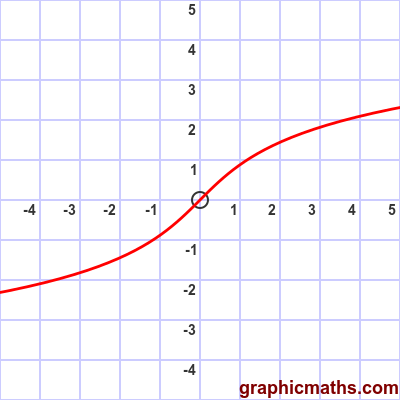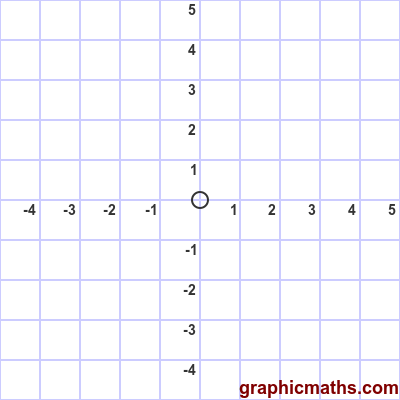# arsinh function

Martin McBride, 2020-02-03
Tags arsinh
Categories hyperbolic functions

The arsinh function is a hyperbolic function. It is the inverse of the sinh, and is also known as the inverse hyperbolic sine function.

## Equation and graph

The arsinh function is defined as the inverse of sinh, ie if:

$$x = \sinh y$$

then:

$$y = \operatorname{arsinh} x$$

There is a also a formula for finding arsinh directly:

$$\operatorname{arsinh} x= \ln{x+{\sqrt {x^{2}+1}}}$$

Here is a graph of the function:## arsinh as inverse of sinh

This animation illustrates the relationship between the sinh function and the arsinh function:The first, blue, curve is the sinh function.

The grey dashed line is the line $y=x$.

The second, red, curve is the arsinh function. As with any inverse function, it is identical to the original function reflected in the line $y=x$.